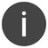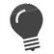# IF

Conditionally execute a statement

 WTSupported in traditional Synergy on Windows WNSupported in Synergy .NET on Windows USupported on UNIX VSupported on OpenVMS
```IF condition statement_1
```

or

```IF condition THEN statement_2
ELSE statement_3
```

Arguments

condition

An expression whose value controls whether the statement is to be executed.

statement_1

A single or compound statement to be executed if ­condition is true.

statement_2

A single or compound statement to be executed if ­condition is true.

statement_3

A single or compound statement to be executed if ­condition is false.

Discussion

The first form of the IF statement conditionally executes a specified statement.

The second form of the IF statement executes one of two statements based on the value of an expression.

In the first form of the IF statement, if condition is true, statement_1 is executed; if condition is false, statement_1 is skipped. In the second form of the IF statement, if condition is true, statement_2 is executed and ­statement_3 is skipped; if condition is false, statement_3 is executed and statement_2 is skipped. In all cases, execution continues with the statement following the IF.

Statement_1, statement_2, statement_3 and the THEN and ELSE keywords can each begin on the next logical line.

By default, Synergy DBL requires that an IF statement with a THEN present must also have an ELSE, and the ELSE belongs to the last THEN in the same lexical level.You can perform IF tests of the following two forms: ```if .NOT. dfield if dfield ```

Examples

Below is an example of the first form of the IF statement.

```clear dflag
display(TTCHN, "Do you want extended screens?")
if (yesno .eq. "Y")
xcall ext_scrns
```

Below is an example of the IF-THEN-ELSE form of the IF statement. In this example, statement1 is processed when answer is equal to Y and choice equals 1, and ­statement3 is processed when answer is not equal to Y.

```if (answer .eq. 'Y') then
if (choice .eq. 1) then
statement1
else
statement2
else
statement3
```

The following is an example of a cascaded IF statement, which is a series of IF-THEN-ELSE statements that have another IF-THEN-ELSE statement following the ELSE keyword:

```    if (a_select .eq. 1) then
result = a_v1 + a_v2
else if (a_select .eq. 2) then
result = a_v1 - a_v2
else if (a_select .eq. 3) then
result = a_v1 * a_v2
else if (a_select .eq. 4)
result = a_v1 / a_v2
```

Although the cascaded IF statement is functionally equivalent to the CASE statement, CASE is more efficient and easier to maintain. See CASE-ENDCASE for more information.To avoid logic errors in nested IF statements, do one of the following: Always use the default Synergy DBL format. Enclose the embedded IF statement in a BEGIN-END compound statement.